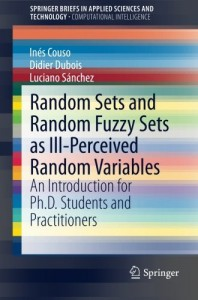The Society for Imprecise Probabilities:
Theories and Applications

# A report on the book ''Random sets and random fuzzy sets as ill-perceived random variables''

Posted on November 11, 2014 by Inés Couso
[ go back to blog ]Random sets and random fuzzy sets as ill-perceived random variables. An introduction for PhD students and practitioners, by Inés Couso, Didier Dubois and Luciano Sánchez. Springer Briefs in Applied Sciences and Technology, subcategories: Springer Briefs in Computational Intelligence. 2014.

The notion of random set has been around for about 50 years, and has been considered rather independently by two sets of prominent authors. There are the works published in the mid-sixties by well-known economists like Aumann and Debreu on the integration of set-valued functions, followed by a full-fledged mathematical development by Kendall and Matheron. Completely apart from this trend is the pioneering work of Dempster who studied upper and lower probabilities induced by a multivalued mapping from a probability space to the range of an attribute of interest. On this basis, Shafer theory of evidence was built. Formally this is an avatar of random set theory.

However in the first “ontic” view,  random sets are considered as precise representations of random complex entities whose outcomes were naturally described by sets of elements. In contrast, Dempster adopts an epistemic stance, and interprets a random set as representing incomplete information on an ill-known random variable. The theory of belief functions adopts a similar understanding to describe a non-additive extension of subjective probabilities that accommodates, again, incomplete information. Besides, the literature contains many papers about fuzzy random variables since the late seventies, where again authors can be shared between ontic and epistemic schools, but the majority of recent works adopts and extend the “ontic” point of view of random sets.

This short book focuses on the use of random sets and fuzzy random variables as natural representations of ill-observed variables or partially known conditional probabilities.  It deals with the use of random sets and fuzzy random variables from an epistemic point of view.  It is closely connected to the possibilistic interpretation of fuzzy sets, suggested by L. A. Zadeh in 1978. Within this context, the relation between possibility measures and families of nested confidence intervals, and their relation with cuts of fuzzy sets was independently studied by Inés Couso and Luciano Sánchez, and Didier Dubois and colleagues during the last decade. The terms ‘‘ontic’’ and ‘‘epistemic’’ fuzzy sets were suggested by D. Dubois and H. Prade in order to distinguish between complex set-valued entities and the representation of partial knowledge about point-valued entities.

The content of this book is based on three chapters, completed with examples and exercises.

The first chapter proposes a genealogy of published works on random sets, fuzzy sets and belief functions since the mid-sixties, explaining the development and the cross-fertilization of the various trends. The second chapter develops the epistemic view of random sets, and their various representations in terms of families of probability functions. It also questions the relevance of classical approaches to random sets that evaluate scalar statistical parameters like variance. The third chapter does the same job with fuzzy random variables understood either as epistemic fuzzy sets of random variables, or as credal sets induced by  conditional possibility functions.

The aim of the book is to emphasize the difference between the ontic and epistemic views of (fuzzy) random sets, so that students or users working with them may choose the correct approach in a given problem. So its contribution is more philosophical and methodological than a formal exposition of the mathematics underlying the subject-matter. It is motivated by the existence of numerous published papers where it is not clear what random sets mean in practice, and where the motivations (typically epistemic: handling imprecision) often look at odds with the proposed approach (often based on the classical statistical approach to random fuzzy sets as a special kind of functional data).